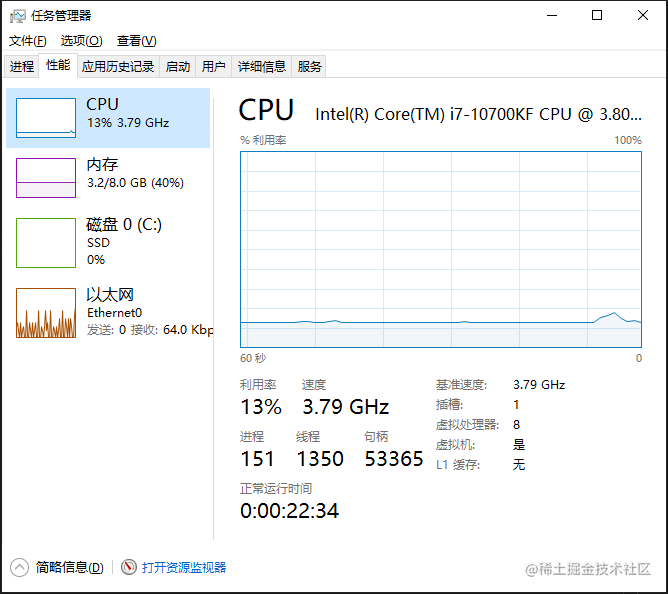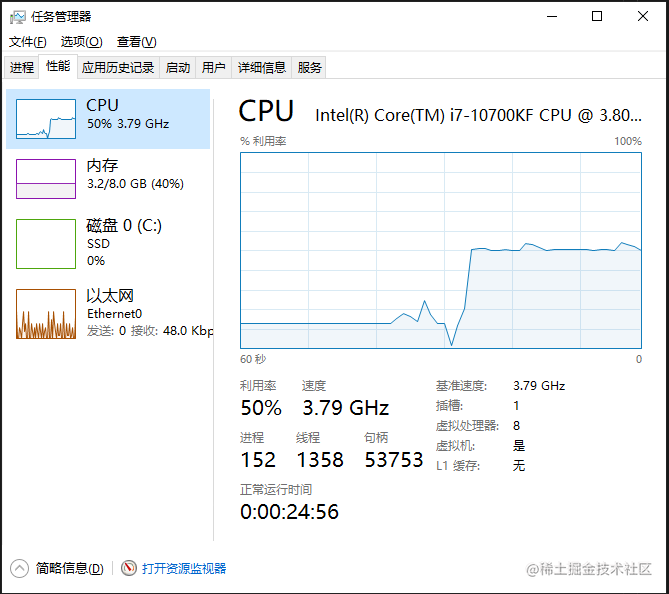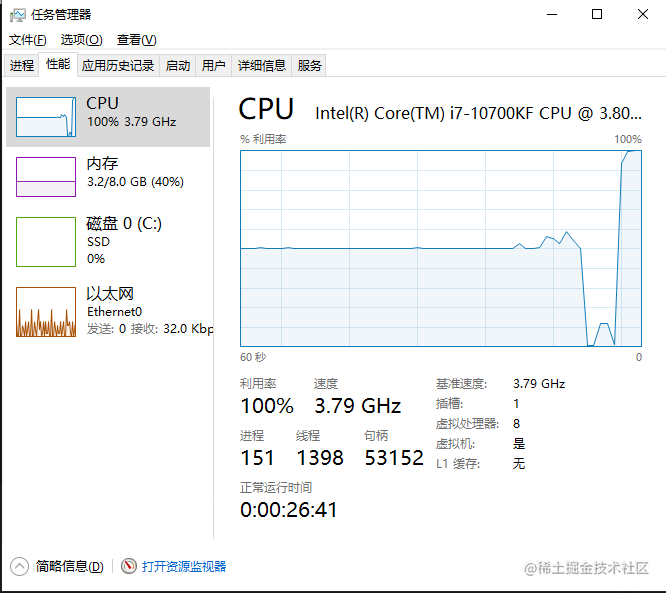# 有什么关系？

CPU的线程指的是同一时间有多少个可以工作的，而操作系统的线程大致可以理解为一个任务能被多少个工作的同时完成。

``````public class MoreLine implements Runnable {

private int count;

@Override
public void run() {
while (true)
this.count++;

}

public int getCount() {
return count;
}

public void setCount(int count) {
this.count = count;
}

public static void main(String[] args) {
t1.start();

// t2.start();

// t3.start();

// t4.start();

// t5.start();

// t6.start();

// t7.start();

// t8.start();
}
}13%和我们想象的基本一致。 这样讲的话我让4个线程工作的话，CPU使用率就能达到50%左右。让我们在来看看。

``````public class MoreLine implements Runnable {

private int count;

@Override
public void run() {
while (true)
this.count++;

}

public int getCount() {
return count;
}

public void setCount(int count) {
this.count = count;
}

public static void main(String[] args) {
t1.start();

t2.start();

t3.start();

t4.start();

// t5.start();

// t6.start();

// t7.start();

// t8.start();
}
}CPU使用率直线上升。